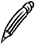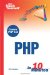# Data Types

Every variable that holds a value also has a data type that defines what kind of value it is holding. The basic data types in PHP are shown in Table 2.2.

##### Table 2.2. PHP Data Types

Data Type

Description

Boolean

A truth value; can be either TRUE or FALSE.

Integer

A number value; can be a positive or negative whole number.

Double (or float)

A floating-point number value; can be any decimal number.

String

An alphanumeric value; can contain any number of ASCII characters.

When you assign a value to a variable, the data type of the variable is also set. PHP determines the data type automatically, based on the value you assign. If you want to check what data type PHP thinks a value is, you can use the gettype function.

Running the following code shows that the data type of a decimal number is double:

` \$value = 7.2; echo gettype(\$value); `

The complementary function to gettype is settype, which allows you to override the data type of a variable. If the stored value is not suitable to be stored in the new type, it will be modified to the closest value possible.

The following code attempts to convert a string value into an integer:

` \$value =  "22nd January 2005"; settype(\$value, "integer"); echo \$value; `

In this case, the string begins with numbers, but the whole string is not an integer. The conversion converts everything up to the first nonnumeric character and discards the rest, so the output produced is just the number 22.Analyzing Data Types In practice, you will not use settype and gettype very often because you will rarely need to alter the data type of a variable. This book covers this topic early on so that you are aware that PHP does assign a data type to every variable.

#### Type Juggling

Sometimes PHP will perform an implicit data type conversion if values are expected to be of a particular type. This is known as type juggling.

For example, the addition operator expects to sit between two numbers. String type values are converted to double or integer before the operation is performed, so the following addition produces an integer result:

` echo 100 + "10 inches"; `

This expression adds 100 and 10, and it displays the result 110.

A similar thing happens when a string operator is used on numeric data. If you perform a string operation on a numeric type, the numeric value is converted to a string first. In fact, you already saw this earlier in this lesson, with the concatenation operatorthe value of \$weight that was displayed was numeric.

The result of a string operation will always be a string data type, even if it looks like a number. The following example produces the result 69, butas gettype shows\$number contains a string value:

` \$number = 6 . 9; echo \$number; echo gettype(6 . 9); `

We will look at the powerful range of operators that are related to numeric and string data types in PHP in Lessons 5, "Working with Numbers," and 6, "Working with Strings."

#### Variable Variables

It is possible to use the value stored in a variable as the name of another variable. If this sounds confusing, the following example might help:

` \$my_age = 21; \$varname = "my_age"; echo "The value of \$varname is \${\$varname}"; `

The output produced is
` The value of my_age is 21 `

Because this string is enclosed in double quotes, a dollar sign indicates that a variable's value should become part of the string. The construct \${\$varname} indicates that the value of the variable named in \$varname should become part of the string and is known as a variable variable.

The braces around \$varname are used to indicate that it should be referenced first; they are required in double-quoted strings but are otherwise optional. The following example produces the same output as the preceding example, using the concatenation operator:

` echo 'The value of ' . \$varname . ' is ' . \$\$varname; `Sams Teach Yourself PHP in 10 Minutes
ISBN: 0672327627
EAN: 2147483647
Year: 2005
Pages: 151
Authors: Chris Newman

Similar book on Amazon

flylib.com © 2008-2017.
If you may any questions please contact us: flylib@qtcs.net